# VBA Course: Arrays

## Arrays

Arrays allow you to store a large number of values unlike variables, which can only store a single value at a time.

We briefly touched on the subject in the lesson on variables, and now we will delve deeper into it.

## Benefits of Arrays

Imagine that in a procedure, you need to store 500 values. If you had to create 500 variables to store all these values, it would quickly become very complicated. However, with an array, the storage and use of these values will be greatly simplified.

The second benefit is execution speed (iterating through an array of data is much faster than iterating through an equivalent range of cells).

Nothing beats an example to better understand...

The first sheet here contains a database of 5000 rows and 3 columns: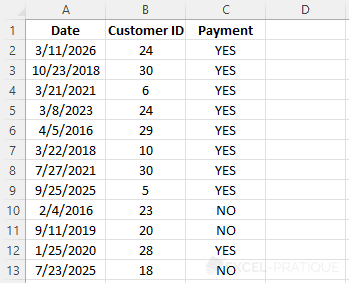The second sheet contains a grid where the YES values will be counted based on years and customers: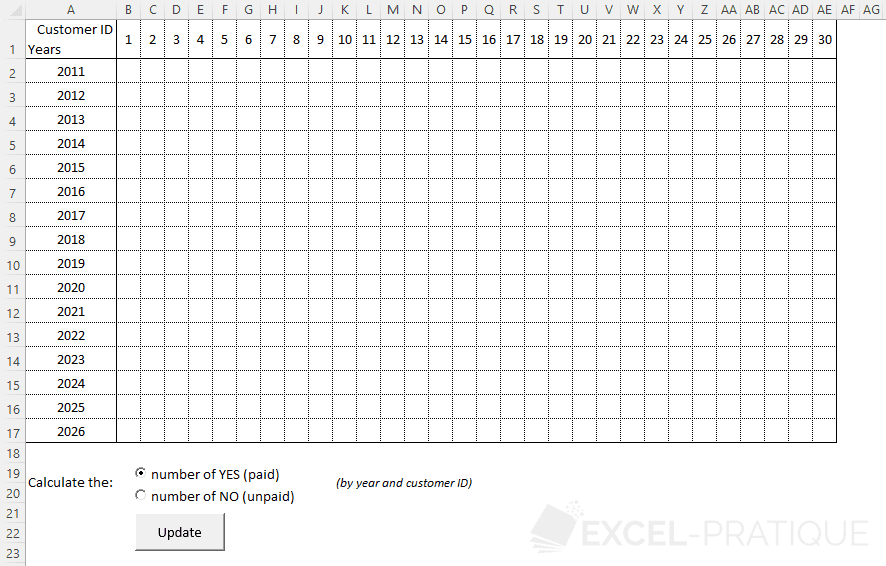In this example, the procedure will loop through the database and count, for each year and customer number, the number of YES values before entering it in the corresponding cell.

Without using an array, it will take Excel 55.27 seconds to execute the procedure: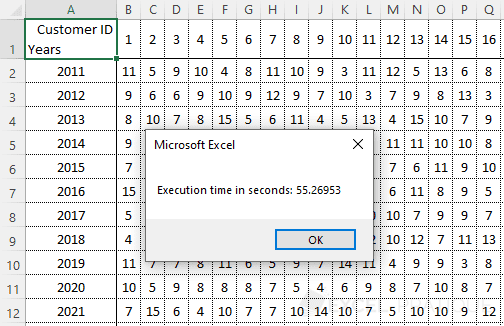By first storing the database in an array and then performing the same calculations (iterating through the array instead of cells), it will only take 0.75 seconds to execute the procedure: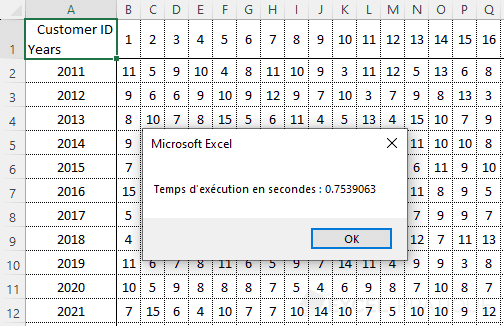If we decide to optimize the procedure by only storing the data with YES values in the array (which represents about 3/4 of the data) and only the years from the dates, 0.23 seconds are sufficient: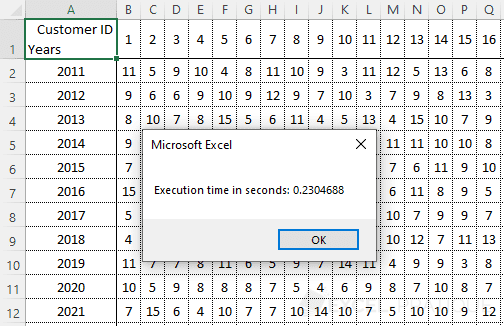In this example, using an array allowed the procedure to be executed approximately 240 times faster.

## Array Declaration

Here are some examples of declarations (if the first two declarations are not clear to you, review this):

``````'Example of declaring a 1-dimensional array
Dim array1(4)

'Example of declaring a 2-dimensional array
Dim array2(6, 1)

'Example of declaring a dynamic array
Dim array3()``````

If you cannot enter fixed values (because it depends on the size of the database, for example), leave the parentheses empty.

You don't need to declare a type (String, Integer, etc.) because, in many cases, it would slow down your procedure.

## Storing Data in an Array

Let's start by storing this data in an array: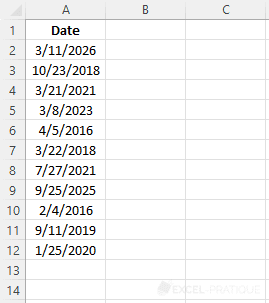Here we want to store 11 x 1 values, so we need to declare a 1-dimensional array:

``Dim myArray(10)``

The array myArray(10) can hold 11 values because remember that the numbering of an array starts at 0.

Each element of the array is then assigned its value:

``````Sub example()

Dim myArray(10)

'Storing values in the array
myArray(0) = Range("A2")
myArray(1) = Range("A3")
myArray(2) = Range("A4")
myArray(3) = Range("A5")
myArray(4) = Range("A6")
myArray(5) = Range("A7")
myArray(6) = Range("A8")
myArray(7) = Range("A9")
myArray(8) = Range("A10")
myArray(9) = Range("A11")
myArray(10) = Range("A12")

End Sub``````

But to avoid all this repetition, using a For loop is highly recommended:

``````Sub example()

Dim myArray(10), i As Integer

'Storing values in the array
For i = 0 To 10
myArray(i) = Range("A" & i + 2)
Next

End Sub``````

Note that you can use and modify each element of the array individually like a variable.

Here's an example with myArray(3), the fourth value of the array:

``````Sub example()

Dim myArray(10), i As Integer

'Storing values in the array
For i = 0 To 10
myArray(i) = Range("A" & i + 2)
Next

'Display 1
MsgBox myArray(3) 'Returns: 3/8/2023

'Modifying one of the values
myArray(3) = Year(myArray(3))

'Display 2
MsgBox myArray(3) 'Returns: 2023

End Sub``````

In this example, the Year function (which returns the year of a date) was used to modify myArray(3).